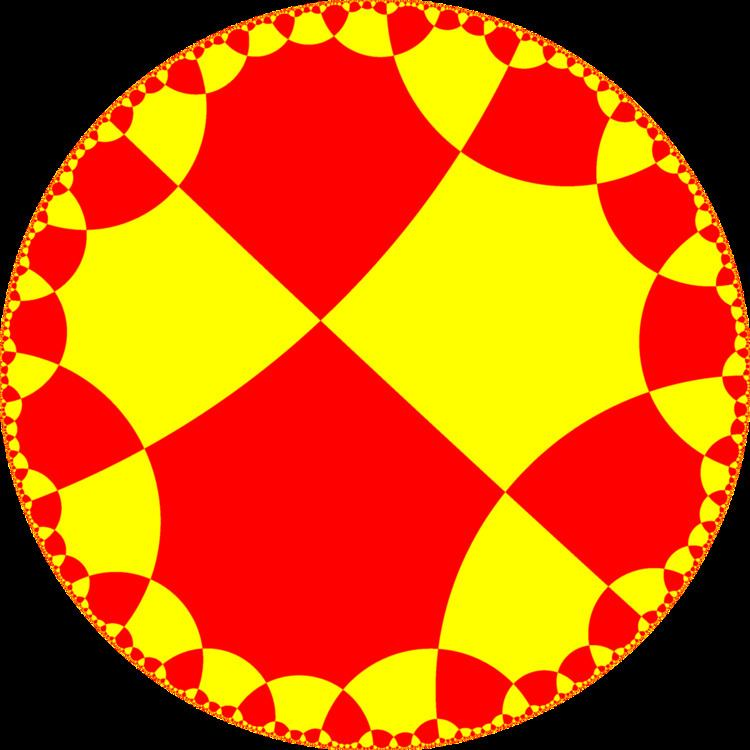# Hexaoctagonal tiling

Updated onIn geometry, the hexaoctagonal tiling is a uniform tiling of the hyperbolic plane.

## Constructions

There are four uniform constructions of this tiling, three of them as constructed by mirror removal from the [8,6] kaleidoscope. Removing the mirror between the order 2 and 4 points, [8,6,1+], gives [(8,8,3)], (*883). Removing the mirror between the order 2 and 8 points, [1+,8,6], gives [(4,6,6)], (*664). Removing two mirrors as [8,1+,6,1+], leaves remaining mirrors (*4343).

## Symmetry

The dual tiling has face configuration V6.8.6.8, and represents the fundamental domains of a quadrilateral kaleidoscope, orbifold (*4343), shown here. Adding a 2-fold gyration point at the center of each rhombi defines a (2*43) orbifold. These are subsymmetries of [8,6].

## References

Similar Topics
Flight to Nowhere
Denis Boytsov
Poornima Bhagyaraj
Topics